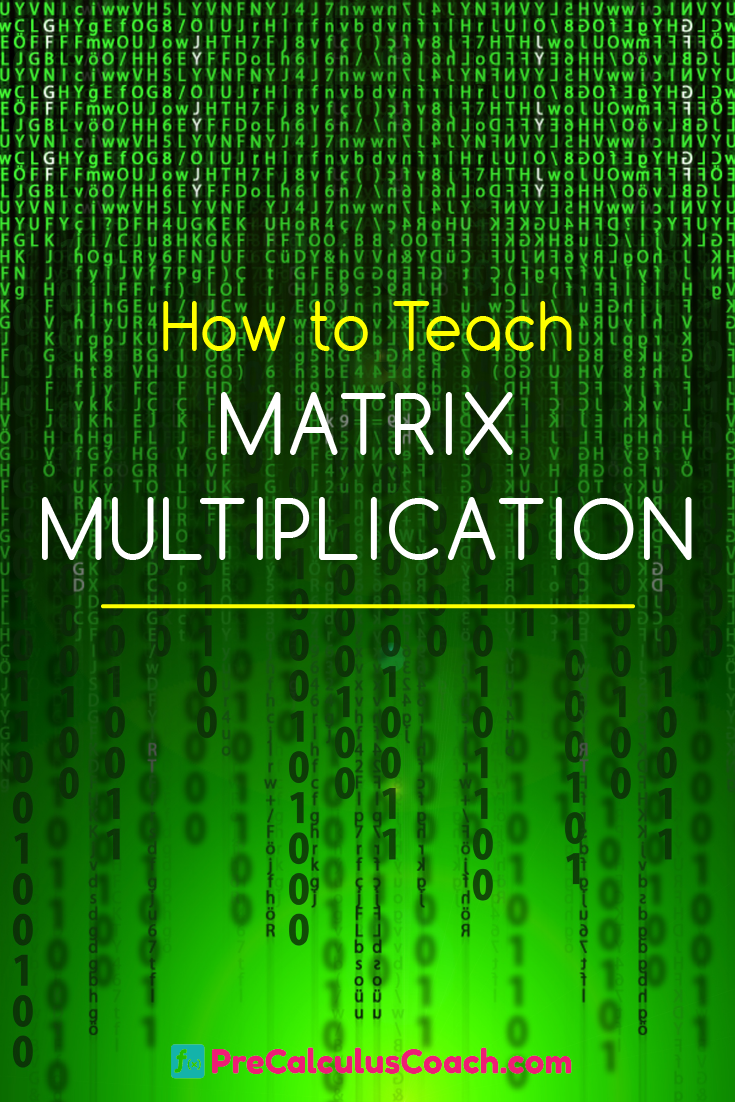# How to Teach Matrix Multiplication

 Would You Rather Listen to the Lesson?The ‘Illuminations” Lessons from the NCTM website are an excellent resource for many mathematics concepts. Math teachers often take the ideas of others and tweak them to suit their level of comfort or match the activity to the ability level of students.

One of the lessons entitled, Computer Animation, is a fun way for students to relate the content of a matrix to something concrete. It also allows students to evaluate their understanding of the concept or to see where mistakes are made.Understanding why or where a mistake is made based on an activity done in class gives students some ownership in the learning process.

It has been said that a person must hear something 17 times before it registers. Using a video from sources such as YouTube or Khan Academy as a second, third, fourth, etc. explanation is helpful to students. Hopefully your students have mastered Adding & Subtracting Matrices by now.

The link below is a link to review how to multiply matrices. The suggested lesson plan below is just that, a suggestion. The material can be used to review multiplication of matrices, put the use of matrices in a contextual format, or serve as an introduction to using a graphing calculator to compute matrices.

## Matrix Multiplication Lesson

The lesson may be something like this:

• Show this video to review how to multiply matrices.
• Give students a copy of just the first diagram provided, the stickman graph.
• Have them construct a matrix where the labeled x coordinates are the top row of the pattern, and the y coordinates are the bottom row of the model.
• If students need practice with multiplying matrices, have them multiply the matrix they devised by the matrices in Problem 2.
• If students are adept at multiplying matrices, teach them to use a calculator to compute the products.
• Have them graph each of the new matrices and describe what happened. If the result is not a reflection, rotation, translation, or dilation of the original, a mistake has been made. You might want to share that information with students after they graph the first new one. Students working in groups can help each other find any errors that are made.

Let students choose one of the other diagrams. Have them find a new matrix and graph it for homework. If they can get one done correctly, they probably have mastered the concept.

## Matrix Multiplication Worksheets - PDFs

6-2 Guided Notes Teacher Edition (Members Only)

6-2 Lesson Plan (Members Only)

6-2 Online Activities (Members Only)

6-2 Video Lesson (Members Only)

## Matrix Multiplication Worksheets, Word Docs & PowerPoints

To gain access to our editable content Join the Pre-Calculus Teacher Community!
Here you will find hundreds of lessons, a community of teachers for support, and materials that are always up to date with the latest standards.